# Tunnel diode oscillator and its application for studying the magnetic penetration depth

The superconducting gap symmetry is a fundamental property with respect to the study of superconductors. Unconventional superconductors normally possess peculiar gap symmetries, which result in distinct behaviors of the superfluid density. A tunnel diode oscillator (TDO) based system was assembled at the CCM to probe the superfluid density. In this device, the stability of the oscillations of the LC circuit and high sensitivity of the measurements, is achieved by using a tunnel diode. By putting a small sample into the coil of the oscillator (see Figure. 1), one can probe tiny changes of the sample’s radio frequency and deduce the magnetic penetration depth.

The resonant frequency of the oscillator is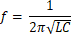(1)

The inductance difference due to the sample magnetic susceptibility is given by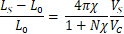(2)

The sample geometry (characterized by R3D) determines the relationship between the susceptibility shift and the change of the penetration depth, where.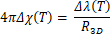(3)

Consequently, the change of the penetration depth from the zero temperature value is proportional to the corresponding change of resonant frequency,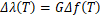(4)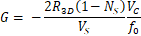(5)

Here the proportionality constant G depends on the system and sample geometry. Finally, the superfluid density can be directly derived from the penetration depth using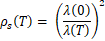(6)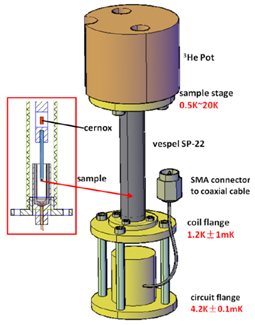Figure.1: Experimental setup of the tunnel diode oscillator on the  cryostat.

The device is designed so that it can be attached to a range of commercial cryostats, allowing for the change of susceptibility to be measured down to the mK range. Moreover, it also allows us to measure changes of the magnetic penetration depth of the order of a few parts per billion. Compared to other measurements such as μSR or SQUID magnetometry, TDO has the simplest design and directly probes the fundamental physical properties, making it an excellent tool for investigating the superconducting gap symmetry.

In the past few years, the TDO method has been efficiently used to measure the penetration depth of more than ten newly-discovered superconductors, and their gap symmetry has been precisely determined.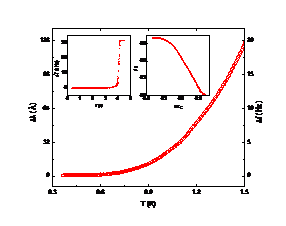Figure.2: Temperature dependence of the penetration depth and superfluid density of a typical BCS superconductor

Since the resonant frequency of the TDO is also sensitive to the surface conductance of a normal metal, we recently extended the application of the TDO technique by combining it with high magnetic field and high pressure measurement. We found this to be a powerful probe for detecting different types of phase transitions,  as well as quantum oscillations.

Subscribe!